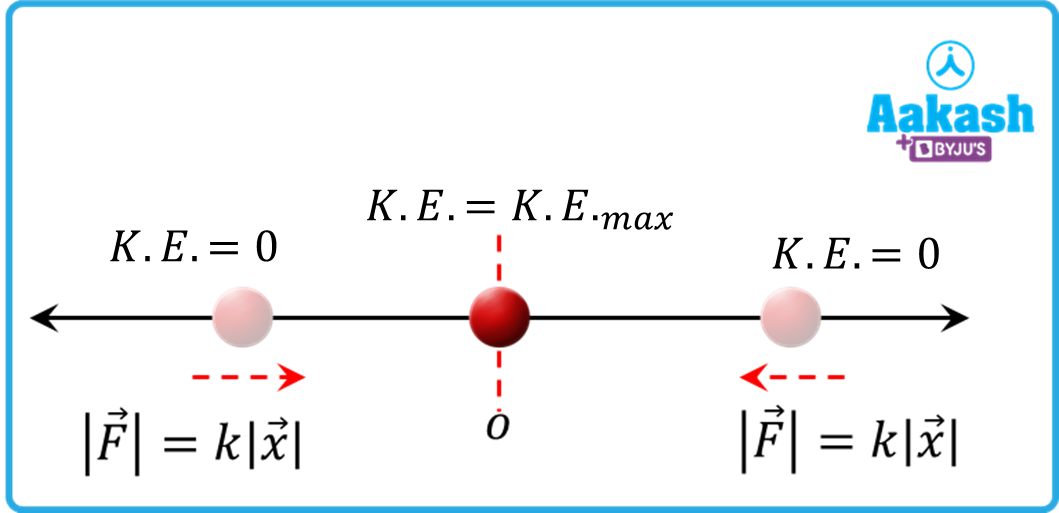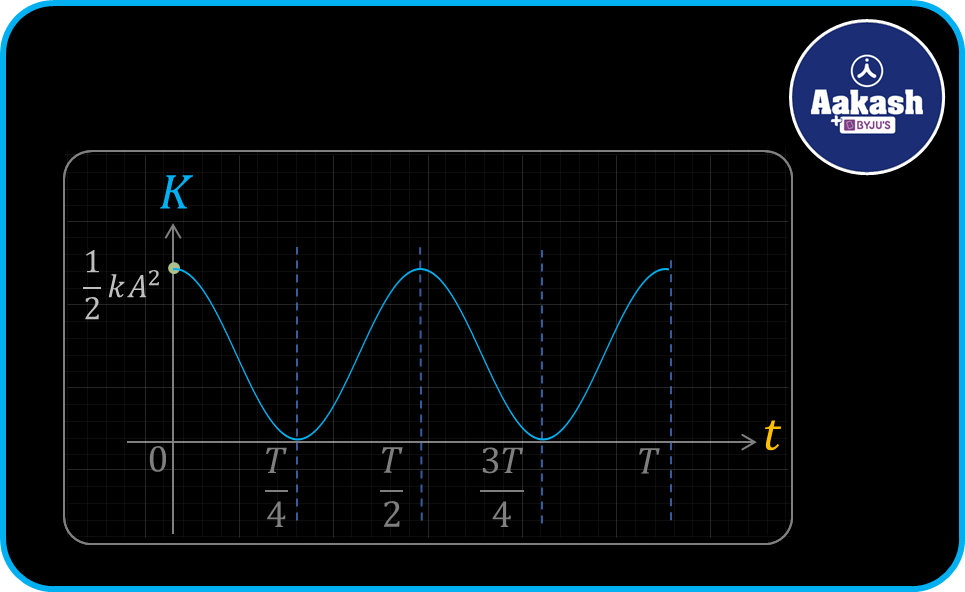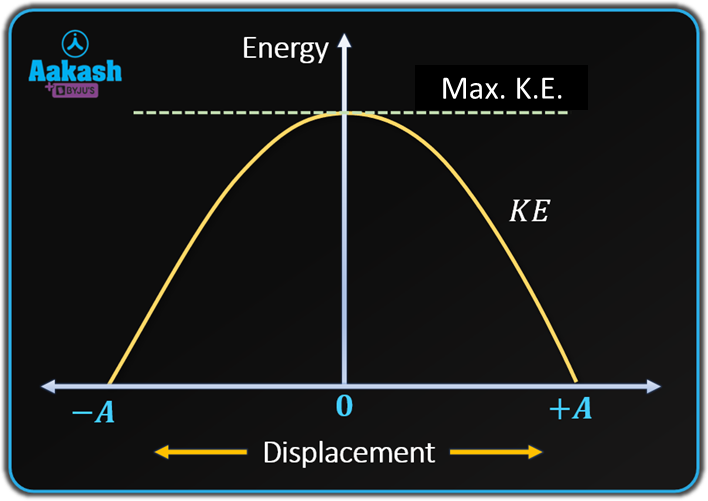• Call Now

1800-102-2727•

Kinetic Energy Formula in SHM - Practice problems,FAQs

Consider two identical cars moving on a road, one belonging to you and the other to your friend. Suddenly the engines of the cars turned off, now they are moving due to their kinetic energies. As the road has friction so the cars will stop after moving some distance. Can you answer which car will stop first? Yes, if we know the kinetic energies of the car then we can compare which car will stop first. The kinetic energy of the car can be calculated using the kinetic energy formula. Let's find out what is the kinetic energy formula for a body in motion !

Table of content

• Kinetic Energy
• Kinetic Energy of a simple harmonic motion
• Kinetic Energy formula of a simple harmonic motion
• Practice problems
• FAQs

## Kinetic Energy

Kinetic energy is a type of energy that a moving object or particle possesses.When work, which involves the transfer of energy, is done on an object by applying a net force, that object acquires kinetic energy. The kinetic energy of a moving object or particle is a function of its mass and velocity of motion. The motion can be in the form of vibration, rotation around an axis, translation (or transit along a path from one location to another), or any combination of these.

A body's translational kinetic energy is equal to half of the product of its mass (m) and it's the square of its velocity (v).

$K.E.=\frac{1}{2}m{v}^{2}$

The unit of kinetic energy is Joule.

## Kinetic Energy of a simple harmonic motion

A simple harmonic motion is an oscillatory motion in which the force is directly proportional to the displacement of the particle from the mean position.Due to the above fact, When a particle executes simple harmonic motion its velocity changes with time and position. When the particle is at its mean position it has maximum velocity. As the kinetic energy is proportional to the square of velocity, so particles have maximum kinetic energy at the mean position. Also when the particle is at extreme positions it has zero velocity so the kinetic energy will be zero . Let's see mathematically how we calculate kinetic energy in simple harmonic motion.

## Kinetic Energy formula of a simple harmonic motion

The formula of the kinetic energy of a simple harmonic motion can be given in two ways - (1) As a function of time and (2) As a function of displacement from the mean position.

• Kinetic energy formula as a function of time

Consider a particle of mass m executing simple harmonic motion with amplitude A and angular frequency , as shown in the above figure. The displacement of the particle at time t is given as,

Also the velocity of the particle can be found by differentiating the above equation w.r.t. time, we get,

The kinetic energy of the particle in motion is given by

$K.E.=\frac{1}{2}m{v}^{2}\dots ..\left(iii\right)$

From equestion (ii) and (iii)

$K.E.=\frac{1}{2}m{\left[A\omega \mathrm{cos}\left(\omega t\right)\right]}^{2}$

where $m{\omega }^{2}=k$ is known as force constant.

This is the formula for kinetic energy as a function of t. From the above equation we can conclude that the kinetic energy of a particle in shm is a function of the square of cos whose maximum value is, $K.E{.}_{max}=\frac{1}{2}k{A}^{2}$.

Hence the graph of the kinetic energy with respect to time is given below,• Kinetic energy formula as a function of displacement from the mean position

Again considering the above particle, executing simple harmonic motion. The velocity of the particle as a function displacement form the mean position is given as,

The kinetic energy of the particle is given by

$K.E.=\frac{1}{2}m{v}^{2}\dots ..\left(ii\right)$

On putting the value of v from equation (i) to equation (ii)

$K.E.=\frac{1}{2}m{\left[\omega \sqrt{{A}^{2}-{x}^{2}}\right]}^{2}$

$K.E.=\frac{1}{2}m{\omega }^{2}\left({A}^{2}-{x}^{2}\right)$

where $m{\omega }^{2}=k$ is known as force constant.

$K.E.=\frac{1}{2}k\left({A}^{2}-{x}^{2}\right)$

This is the formula for kinetic energy in terms of displacement x from the mean position. We can conclude from the above equation that kinetic energy is a function of the square of displacement from the mean position. At x=0 (i.e. at mean position) the kinetic energy will be maximum $K.E{.}_{max}=\frac{1}{2}k{A}^{2}$. And x=A (i.e. at an extreme position) the kinetic energy will be zero.

The graph of kinetic energy w.r.t displacement x is shown in the figure below,## Practice problems

Q1. A block of mass 10 kg moving with the velocity 8 m/s. What is the block's kinetic energy?

A. Given m=10 kg and $v=8\frac{m}{s}$

The kinetic energy of a particle in motion is,

$K.E.=\frac{1}{2}m{v}^{2}$

$K.E.=\frac{1}{2}×10×{8}^{2}$

K.E.=320 J

Hence the kinetic energy of the block is 320 J.

Q2. A particle of mass 15 kg is placed at the origin. It starts oscillation along the x-axis between

- 5 cm to + 5 cm with the angular frequency of$\pi \frac{rad}{s}$. What is the kinetic energy of the particle at $t=\frac{1}{3}s$.

Amplitude A=5 cm=0.05 m

angular frequency $\omega =\pi \frac{rad}{s}$

Time $t=\frac{1}{3}s$

The particle's kinetic energy is determined by,

K.E.=0.046 J

Hence the kinetic energy of the particle is 0.046 J

Q3. A block of mass 5 kg is attached with a spring of force constant 100 N/m. When it is given some velocity, it starts executing simple harmonic motion with an amplitude of 10 cm. What is the kinetic energy of the particle when it is at a distance 5 cm from the mean position.

force constant $k=100\frac{N}{m}$

Amplitude A=10 cm=0.1 m

x=5 cm=0.05 m

The kinetic energy of the particle is

$K.E.=\frac{1}{2}k\left({A}^{2}-{x}^{2}\right)$

$K.E.=\frac{1}{2}×100×\left({0.1}^{2}-{0.05}^{2}\right)$

K.E.=0.375 J

Hence the kinetic energy of the block is 0.375 J

Q4. What is the maximum and minimum kinetic energy of a system (mass 20 kg) in simple harmonic motion with angular frequency and amplitude 7.5 cm.

Amplitude A=7.5 cm=0.75 m

angular frequency =

The simple harmonic motion of the kinetic energy is maximum at the mean position and is given as,

The minimum kinetic energy is at the extreme position which is equal to zero.

Hence the maximum kinetic energy is 124.9 J and minimum kinetic energy is zero.

## FAQs

Q1. What is the nature of the graph of kinetic energy of a particle in SHM w.r.t displacement from mean position?
The kinetic energy is the function of the square of displacement from the mean position, so the graph is parabolic in nature.

Q2. How does the kinetic energy of a system become zero at an extreme position in SHM?
At the extreme position, all the kinetic energy is converted into potential energy of the system. Due to this reason the kinetic energy becomes zero at extreme position.

Q3. The total energy of a system in simple harmonic motion is constant, true or false?
True, the total energy remains constant. And it is equal to the maximum kinetic energy of the system.

Q4. At what position the kinetic energy will be half of the maximum value in simple harmonic motion.

Answer. Given $K.E.=\frac{1}{2}K.E{.}_{max}$

Hence at distance $\frac{A}{\sqrt{2}}$ the kinetic energy will be half of its maximum value.Talk to our expert
Resend OTP Timer =
By submitting up, I agree to receive all the Whatsapp communication on my registered number and Aakash terms and conditions and privacy policy